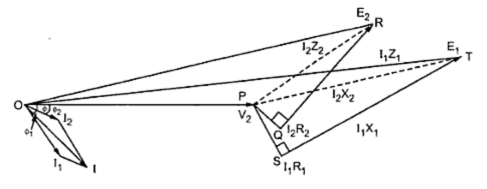### Parallel Operation of Transformers with Unequal Voltage Ratios

Parallel Operation of Transformers with Unequal Voltage Ratios
Now we will consider the case of two transformers working in parallel and having unequal voltage ratio. This is shown in the Fig. 1.
The voltage ratios of the two transformers are not equal. The parallel operation under this case is still possible. But as seen previously there would be a circulating current under no load condition.Fig. 1
Let us consider voltage ratio of transformer 1 is slightly more than 2. So that induced e.m.f.. E1 is greater than E2. Thus the resultant terminal voltage will be E1 - E2 which will cause a circulating current under no load condition.
Ic = (E1 - E2)/(Z1 + Z2 )
From the circuit diagram we have,
E1 = V2 + I1 Z1
E2 = V2 + I2 Z2
Also,                    IL = I1 + I2
V2 = IL ZL = ( II + I2 ) ZL
E1 =(I1 + I2) ZL + II Z1                   .........(a)
E2 =(I1 + I2 ) ZL + I2 Z2               .......(b)
Subtracting equations (a) and (b) we have,
E1 - E2 = I1 Z1 - I2 Z2
I1 = ((E1 - E2) + I2 Z2) /Z1
Subtracting this value in equation (b),

...                       I2 = (E2 Z1 - (E1 - E2)ZL) / (Z1 Z2 + ZL (Z1 + Z2))
Similarly,             I1 = (E1 Z2 + (E1 - E2)ZL)/ (Z1 Z2 + ZL (Z1 + Z2))
Adding the above equations,
I1 + I2 = (E1 Z2 + E2 Z1) / (Z1 Z2 + ZL (Z1 + Z2))           .............(c)
But                     IL = I1 + I2
Load voltage,     V2 = IL ZL
If impedances Z1 and Z2 are small in comparision with load impedance ZL then product Z1 Z2 may be neglected so we get,
But we know that
(E1 - E2) / (Z1 + Z2) = Ic
Key Point : This circulating current Ic adds to I1 but subtracts from I2. Hence transformer 1 gets overloaded. The transformers will not share the load according to their ratings.
The phasor diagram is shown in the Fig. 2.Fig. 2
The two transformers will operate at different power factor Φ1 and Φ2 are the power factor angles of these two transformers whereas Φ is the combined p.f. angle.
Here EA and EB have the same phase but there may be some phase difference between the two due to some difference of internal connection as for the connection in parallel of a Star/Star and a Star/Delta 3 phase transformers.
Key Point : While solving the problems on parallel operation of transformers it is convenient to work with numerical values of impedances instead of percentage values.